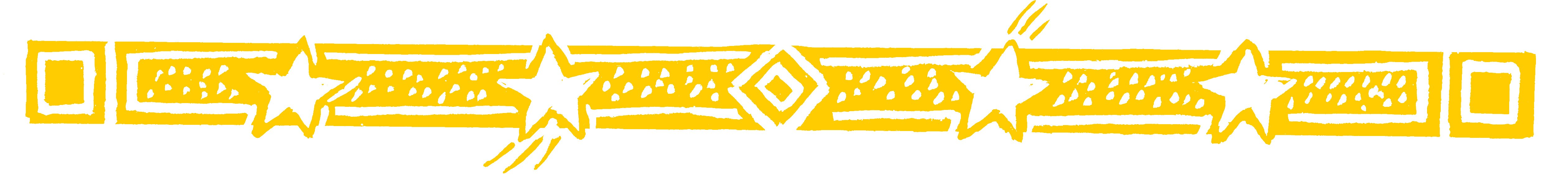Teacher - show your class the easy way to add and subtract fractions.

Use VERTICALLY AND CROSSWISE to write the answer straight down!

•Multiply crosswise and add to get the top of the answer:
2 x 5 = 10 and 1 x 3 = 3. Then 10 + 3 = 13.
The bottom of the fraction is just 3 x 5 = 15.
You multiply the bottom numbers together.

So:

•Subtracting is just as easy: multiply crosswise as before, but then subtract:

•Question Comment !
 Tutorial Title Question Title No Questions 0 Questions Asked 0 Skipped Questions 0 Correctly Answered 0 Wrongly Answered 0 Total Question Attempts 0 Current Question Attempts 0

Skip Question

Reset Test

Halt Test

 No Questions 0 Questions Asked 0 Skipped Questions 0 Correctly Answered 0 Wrongly Answered 0 Total Question Attempts 0

Time Taken

CloseShow Your Class... nature is the
realisation of the
simplest conceivable
mathematical ideas
EINSTEIN